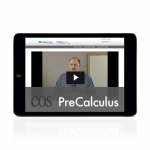## PreCalculus ProductsIncludes everything you need for a successful experience with PreCalculus:

• Instruction Manual with complete solutions
• Instruction DVD
• Student Workbook
• Tests booklet

Order HereThe PreCalculus Student Pack contains the Student Workbook with lesson-by-lesson worksheets and review pages and the PreCalculus Tests.

Order HereThe PreCalculus Instruction Pack contains the instruction manual with lesson-by-lesson instructions and detailed solutions, and the DVD with lesson-by-lesson video instruction..

Order HereLifetime access to PreCalculus streaming instruction videos and online Instruction Manual, lesson and test solutions, and other online resources from any browser. Access begins at time of online purchase or redemption of access code. Note: Digital Packs do not include Student Workbook or Tests..

Order Here

# PreCalculus

### Trigonometry, identities, polar equations, logarithms, sequences, limits and other topics to prepare for calculus.

Major Concepts and Skills Include:

• Understanding and working with trigonometric ratios, their reciprocals, and their inverses
• Working with trigonometric expressions and identities
• Understanding and working with polar coordinates and radian measure
• Graphing and analyzing trigonometric functions
• Understanding functions, limits, domain, and range
• Working with Arithmetic and Geometric Series and Sequences

• Understanding and applying the Laws of Sines and Cosines
• Understanding and applying the Sum, Difference, Double-Angle, and Half-Angle Identities
• Proving trigonometric identities
• Working with vectors and adding linear functions
• Understanding and working with logarithms
• Working with Euler’s number and natural logarithms

## Sample Lesson

• Pythagorean Theorem
• Special Right Triangles (30°- 60°- 90° and 45°- 45°- 90°)
• Trigonometric Ratios
• Inverse Trigonometric Ratios
• Interpreting the Trigonometry Tables
• Using the Trig. Table to Solve for the Unknown
• Using a Calculator and Arc Functions
• Angles of Elevation and Depression
• Angles less than 0°
• Angles greater than 360°
• Reference Angles
• Cofunctions
• Negative Angle Relationships
• Proving Trigonometric Identities
• Sum and Difference Identities
• Double-Angle and Half-Angle Identities
• Law of Sines
• Law of Cosines
• Ambiguity in the Law of Sines
• Polar Coordinates and Rectangular Coordinates
• Polar Equations and Polar Graphs
• Vectors
• Functions: Relation, Domain, and Range
• Graphing the Sine and Cosine Functions
• Graphing the Secant and Cosecant Functions
• Graphing the Tangent and Cotangent Functions
• Logarithms
• Arithmetic Sequences and Series
• Geometric Sequences and Series
• Proof of Cos (A – B) = Cos A Cos B + Sin A Sin B
• Finding the Area of a Triangle Trigonometrically
• Interpolation
• Natural Logarithms
• Equations with Absolute Value
• Inequalities with Absolute Value
• Limits

## View PreCalculus Sample Lesson PDFPreCalculus Digital Pack - Canadian EditionLifetime access to PreCalculus streaming instruction videos and online Instruction Manual, lesson and test solutions, and other online resources from any browser. Access begins at time of online purchase or redemption of access code. Note: Digital Packs do not include Student Workbook or Tests.\$98.00PreCalculus Instruction Pack - Canadian EditionThe PreCalculus Instruction Pack contains the instruction manual with lesson-by-lesson instructions and detailed solutions, and the DVD with lesson-by-lesson video instruction.\$127.00PreCalculus Student Pack - Canadian EditionThe PreCalculus Student Pack contains the Student Workbook with lesson-by-lesson worksheets and review pages and the PreCalculus Tests.\$63.50PreCalculus Universal Set - Canadian EditionIncludes everything you need for a successful experience with PreCalculus: